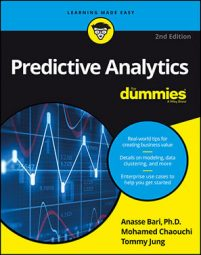##### Predictive Analytics For DummiesThe random forest model is an ensemble model that can be used in predictive analytics; it takes an ensemble (selection) of decision trees to create its model. The idea is to take a random sample of weak learners (a random subset of the training data) and have them vote to select the strongest and best model. The random forest model can be used for either classification or regression. In the following example, the random forest model is used to classify the Iris species.

This code listing will load the `iris` dataset into your session:

`>>> from sklearn.datasets import load_iris`

`>>> iris = load_iris()`

## Creating an instance of the classifier

The following two lines of code create an instance of the classifier. The first line imports the random forest library. The second line creates an instance of the random forest algorithm:

`>>> from sklearn.ensemble import RandomForestClassifier`

`>>> rf = RandomForestClassifier(n_estimators=15,`

`random_state=111)`

The `n_estimators` parameter in the constructor is a commonly used tuning parameter for the random forest model. The value is used to build the number of trees in the forest. It's generally between 10 and 100 percent of the dataset, but it depends on the data you're using. Here, the value is set at 15, which is 10 percent of the data. Later, you will see that changing the parameter value to 150 (100 percent) produces the same results.

The n`_estimators` is used to tune model performance and overfitting. The greater the value, the better the performance but at the cost of overfitting. The smaller the value, the higher the chances of not overfitting but at the cost of lower performance. Also, there is a point where increasing the number will generally degrade in accuracy improvement and may dramatically increase the computational power needed. The parameter defaults to 10 if it is omitted in the constructor.

## Running the training data

You'll need to split the dataset into training and test sets before you can create an instance of the random forest classifier. The following code will accomplish that task:

`>>> from sklearn import cross_validation`

`>>> X_train, X_test, y_train, y_test =`

`cross_validation.train_test_split(iris.data,`

`iris.target, test_size=0.10, random_state=111)`

`>>> rf = rf.fit(X_train, y_train)`

• Line 1 imports the library that allows you to split the dataset into two parts.
• Line 2 calls the function from the library that splits the dataset into two parts and assigns the now-divided datasets to two pairs of variables.
• Line 3 takes the instance of the random forest classifier you just created,then calls the fit method to train the model with the training dataset.

## Running the test data

In the following code, the first line feeds the test dataset to the model, then the third line displays the output:

`>>> predicted = rf.predict(X_test)`

`>>> predicted`

`array([0, 0, 2, 2, 2, 0, 0, 2, 2, 1, 2, 0, 1, 2, 2])`

## Evaluating the model

You can cross-reference the output from the prediction against the `y_test` array. As a result, you can see that it predicted two test data points incorrectly. So the accuracy of the random forest model was 86.67 percent.

Here's the code:

`>>> from sklearn import metrics`

`>>> predicted`

`array([0, 0, 2, 2, 2, 0, 0, 2, 2, 1, 2, 0, 1, 2, 2])`

`>>> y_test`

`array([0, 0, 2, 2, 1, 0, 0, 2, 2, 1, 2, 0, 2, 2, 2])`

`>>> metrics.accuracy_score(y_test, predicted)`

`0.8666666666666667 # 1.0 is 100 percent accuracy`

`>>> predicted == y_test`

`array([ True, True, True, True, False, True, True,`

`True, True, True, True, True, False, True,`

`True], dtype=bool)`

How does the random forest model perform if you change the `n_estimators` parameter to 150? It looks like it won’t make a difference for this small dataset. It produces the same result:

`>>> rf = RandomForestClassifier(n_estimators=150,`

`random_state=111)`

`>>> rf = rf.fit(X_train, y_train)`

`>>> predicted = rf.predict(X_test)`

`>>> predicted`

`array([0, 0, 2, 2, 2, 0, 0, 2, 2, 1, 2, 0, 1, 2, 2])`